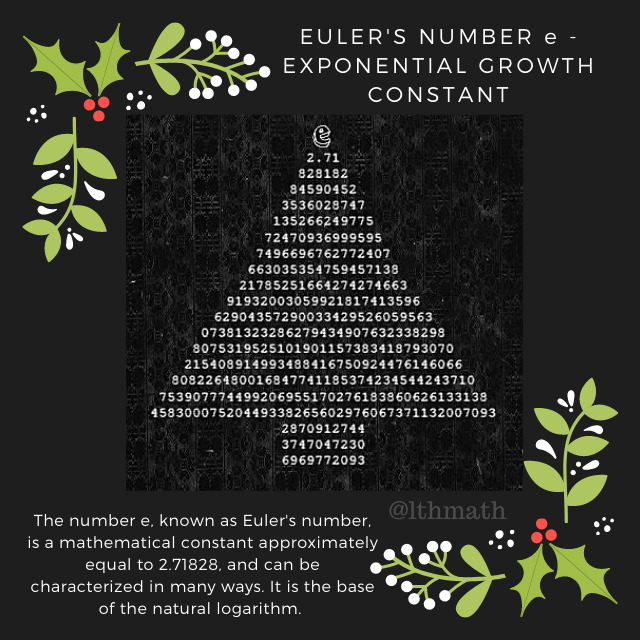Search

# Day 14 | LThMath Advent Calendar

In today's post I wanted to share a little creative way of showing some digits of e. The number e, also known as Euler's number, is a mathematical constant approximately equal to 2.71828, and can be characterized in many ways. When used as the base for a logarithm, we call that logarithm the natural logarithm and write it as lnx. This is another interesting mathematical constants.## General Information

The (natural) exponential function f(x) = e^x is the unique function f that equals its own derivative and satisfies the equation f(0) = 1; hence one can also define e as f(1). The natural logarithm, or logarithm to base e, is the inverse function to the natural exponential function. The natural logarithm of a number k > 1 can be defined directly as the area under the curve y = 1/x between x = 1 and x = k, in which case e is the value of k for which this area equals one.

e is sometimes called Euler's number, after the Swiss mathematician Leonhard Euler (not to be confused with γ, the Euler–Mascheroni constant, sometimes called simply Euler's constant), or Napier's constant. However, Euler's choice of the symbol e is said to have been retained in his honor. The constant was discovered by the Swiss mathematician Jacob Bernoulli while studying compound interest.A little bit of self promotion in here, I have used this design to create a little design that you can add to different items, such as T-shirts, hoddies, mugs, tote bags and a little sticker. If you enjoy the design, take a look at our Teespring shop.